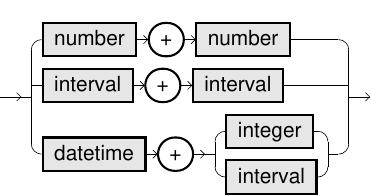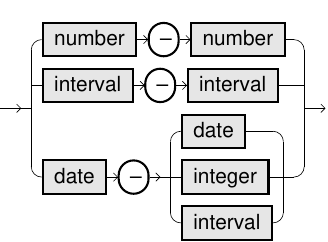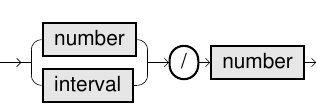# Arithmetic Operators

Arithmetic operators perform mathematical operations on two values or more.

## Syntax

+ operator::=- operator::=* operator::=/ operator::=## Usage Notes

For '+' operator:

• If you add a decimal value to a timestamp, the result depends on the session/system parameter TIMESTAMP_ARTITHMETIC_BEHAVIOR:
• When TIMESTAMP_ARTITHMETIC_BEHAVIOR = 'INTERVAL' - the decimal is rounded to an integer, and a certain number of full days are added (this is similar to function ADD_DAYS).
• When TIMESTAMP_ARTITHMETIC_BEHAVIOR = 'DOUBLE' - the fraction of days are added (hours, minutes, ...).
• When adding the number of years or months (for example, INTERVAL '3' MONTH) on a date whose day is the last day of the month, then the last day of the resulting month is returned (similar to ADD_MONTHS).

For '-' operator

• The difference between two datetime values depends on the session/system parameter TIMESTAMP_ARITHMETIC_BEHAVIOR:
• TIMESTAMP_ARITHMETIC_BEHAVIOR = 'INTERVAL' - the result is an interval (similar to function DAYS_BETWEEN).
• TIMESTAMP_ARITHMETIC_BEHAVIOR = 'DOUBLE' - the result is a double.
• The difference of a date and a decimal is similar to the + operator with a negative value, thus subtracting days instead of adding them.
• The difference between two interval values return an interval.
• When subtracting the number of years or months (for example, INTERVAL '3' MONTH) on a date whose day is the last day of the month, then the last day of the resulting month is returned (similar to ADD_YEARS and ADD_MONTHS).
###### Example
``SELECT 1000 + 234                                           ADD1,DATE '2000-10-05' + 1                                       ADD2,DATE '2009-01-05' + INTERVAL '1-2' YEAR TO MONTH            ADD3,100000 - 1                                                  SUB1,DATE '2000-10-05' - 1                                       SUB2,DATE '2009-01-05' - INTERVAL '2' MONTH                      SUB3,100 * 123                                                   MUL,100000 / 10                                                 DIV;``